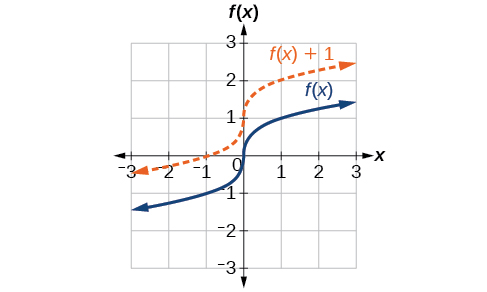# 1.5 Transformation of functions

 Page 1 / 22
In this section, you will:
• Graph functions using vertical and horizontal shifts.
• Graph functions using reflections about the $\text{\hspace{0.17em}}x$ -axis and the $\text{\hspace{0.17em}}y$ -axis.
• Determine whether a function is even, odd, or neither from its graph.
• Graph functions using compressions and stretches.
• Combine transformations.

We all know that a flat mirror enables us to see an accurate image of ourselves and whatever is behind us. When we tilt the mirror, the images we see may shift horizontally or vertically. But what happens when we bend a flexible mirror? Like a carnival funhouse mirror, it presents us with a distorted image of ourselves, stretched or compressed horizontally or vertically. In a similar way, we can distort or transform mathematical functions to better adapt them to describing objects or processes in the real world. In this section, we will take a look at several kinds of transformations.

## Graphing functions using vertical and horizontal shifts

Often when given a problem, we try to model the scenario using mathematics in the form of words, tables, graphs, and equations. One method we can employ is to adapt the basic graphs of the toolkit functions to build new models for a given scenario. There are systematic ways to alter functions to construct appropriate models for the problems we are trying to solve.

## Identifying vertical shifts

One simple kind of transformation involves shifting the entire graph of a function up, down, right, or left. The simplest shift is a vertical shift , moving the graph up or down, because this transformation involves adding a positive or negative constant to the function. In other words, we add the same constant to the output value of the function regardless of the input. For a function $\text{\hspace{0.17em}}g\left(x\right)=f\left(x\right)+k,\text{\hspace{0.17em}}$ the function $\text{\hspace{0.17em}}f\left(x\right)\text{\hspace{0.17em}}$ is shifted vertically $\text{\hspace{0.17em}}k\text{\hspace{0.17em}}$ units. See [link] for an example.Vertical shift by   k = 1   of the cube root function   f ( x ) = x 3 .

To help you visualize the concept of a vertical shift, consider that $\text{\hspace{0.17em}}y=f\left(x\right).\text{\hspace{0.17em}}$ Therefore, $\text{\hspace{0.17em}}f\left(x\right)+k\text{\hspace{0.17em}}$ is equivalent to $\text{\hspace{0.17em}}y+k.\text{\hspace{0.17em}}$ Every unit of $\text{\hspace{0.17em}}y\text{\hspace{0.17em}}$ is replaced by $\text{\hspace{0.17em}}y+k,\text{\hspace{0.17em}}$ so the $\text{\hspace{0.17em}}y\text{-}$ value increases or decreases depending on the value of $\text{\hspace{0.17em}}k.\text{\hspace{0.17em}}$ The result is a shift upward or downward.

## Vertical shift

Given a function $f\left(x\right),$ a new function $g\left(x\right)=f\left(x\right)+k,$ where $\text{\hspace{0.17em}}k$ is a constant, is a vertical shift    of the function $f\left(x\right).$ All the output values change by $k$ units. If $k$ is positive, the graph will shift up. If $k$ is negative, the graph will shift down.

## Adding a constant to a function

To regulate temperature in a green building, airflow vents near the roof open and close throughout the day. [link] shows the area of open vents $\text{\hspace{0.17em}}V\text{\hspace{0.17em}}$ (in square feet) throughout the day in hours after midnight, $\text{\hspace{0.17em}}t.\text{\hspace{0.17em}}$ During the summer, the facilities manager decides to try to better regulate temperature by increasing the amount of open vents by 20 square feet throughout the day and night. Sketch a graph of this new function.

We can sketch a graph of this new function by adding 20 to each of the output values of the original function. This will have the effect of shifting the graph vertically up, as shown in [link] .

Notice that in [link] , for each input value, the output value has increased by 20, so if we call the new function $\text{\hspace{0.17em}}S\left(t\right),$ we could write

$S\left(t\right)=V\left(t\right)+20$

This notation tells us that, for any value of $\text{\hspace{0.17em}}t,S\left(t\right)\text{\hspace{0.17em}}$ can be found by evaluating the function $\text{\hspace{0.17em}}V\text{\hspace{0.17em}}$ at the same input and then adding 20 to the result. This defines $\text{\hspace{0.17em}}S\text{\hspace{0.17em}}$ as a transformation of the function $\text{\hspace{0.17em}}V,\text{\hspace{0.17em}}$ in this case a vertical shift up 20 units. Notice that, with a vertical shift, the input values stay the same and only the output values change. See [link] .

 $t$ 0 8 10 17 19 24 $V\left(t\right)$ 0 0 220 220 0 0 $S\left(t\right)$ 20 20 240 240 20 20

a colony of bacteria is growing exponentially doubling in size every 100 minutes. how much minutes will it take for the colony of bacteria to triple in size
I got 300 minutes. is it right?
Patience
no. should be about 150 minutes.
Jason
It should be 158.5 minutes.
Mr
ok, thanks
Patience
what is the importance knowing the graph of circular functions?
can get some help basic precalculus
What do you need help with?
Andrew
how to convert general to standard form with not perfect trinomial
can get some help inverse function
ismail
Rectangle coordinate
how to find for x
it depends on the equation
Robert
whats a domain
The domain of a function is the set of all input on which the function is defined. For example all real numbers are the Domain of any Polynomial function.
Spiro
foci (–7,–17) and (–7,17), the absolute value of the differenceof the distances of any point from the foci is 24.
difference between calculus and pre calculus?
give me an example of a problem so that I can practice answering
x³+y³+z³=42
Robert
dont forget the cube in each variable ;)
Robert
of she solves that, well ... then she has a lot of computational force under her command ....
Walter
what is a function?
I want to learn about the law of exponent
explain this
what is functions?
A mathematical relation such that every input has only one out.
Spiro
yes..it is a relationo of orders pairs of sets one or more input that leads to a exactly one output.
Mubita
Is a rule that assigns to each element X in a set A exactly one element, called F(x), in a set B.
RichieRich
If the plane intersects the cone (either above or below) horizontally, what figure will be created?

#### Get Jobilize Job Search Mobile App in your pocket Now!ByBy OpenStaxBy Brooke DelaneyBy Sandhills MLTBy Jams KaloBy John GabrieliBy Michael SagBy OpenStaxBy OpenStaxBy Madison ChristianBy OpenStax